International
Tables for
Crystallography
Volume B
Reciprocal space
Edited by U. Shmueli

International Tables for Crystallography (2006). Vol. B. ch. 2.5, p. 285   | 1 | 2 |

## Section 2.5.2.10. Electron diffraction in electron microscopes

J. M. Cowleya

#### 2.5.2.10. Electron diffraction in electron microscopes

| top | pdf |

Currently most electron-diffraction patterns are obtained in conjunction with images, in electron microscopes of one form or another, as follows.

 (a) Selected-area electron-diffraction (SAED) patterns are obtained by using intermediate and projector lenses to form an image of the diffraction pattern in the back-focal plane of the objective lens (Fig. 2.5.2.2).The area of the specimen from which the diffraction pattern is obtained is defined by inserting an aperture in the image plane of the objective lens. For parallel illumination of the specimen, sharp diffraction spots are produced by perfect crystals. A limitation to the area of the specimen from which the diffraction pattern can be obtained is imposed by the spherical aberration of the objective lens. For a diffracted beam scattered through an angle α, the spread of positions in the object for which the diffracted beam passes through a small axial aperture in the image plane is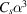, e.g. for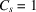mm,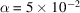rad (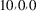reflection from gold for 100 keV electrons),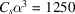Å, so that a selected-area diameter of less than about 2000 Å is not feasible. For higher voltages, the minimum selected-area diameter decreases with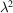if the usual assumption is made thatincreases for higher-voltage microscopes so that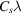is a constant. (b) Convergent-beam electron-diffraction (CBED) patterns are obtained when an incident convergent beam is focused on the specimen, as in an STEM instrument or an STEM attachment for a conventional TEM instrument. For a large, effectively incoherent source, such as a conventional hot-filament electron gun, the intensities are added for each incident-beam direction. The resulting CBED pattern has an intensity distribution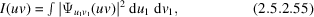where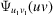is the Fourier transform of the exit wave at the specimen for an incident-beam direction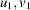. (c) Coherent illumination from a small bright source such as a field emission gun may be focused on the specimen to give an electron probe having an intensity distribution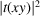and a diameter equal to the STEM dark-field image resolution [equation (2.5.2.47)] of a few Å. The intensity distribution of the resulting microdiffraction pattern is then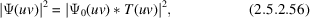where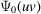is the Fourier transform of the exit wave at the specimen. Interference occurs between waves scattered from the various incident-beam directions. The diffraction pattern is thus an in-line hologram as envisaged by Gabor (1949). (d) Diffraction patterns may be obtained by using an optical diffractometer (or computer) to produce the Fourier transform squared of a small selected region of a recorded image. The optical diffraction-pattern intensity obtained under the ideal conditions specified under equation (2.5.2.54)is given, in the case of weak phase objects, by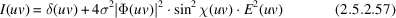or, more generally, by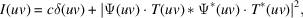where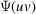is the Fourier transform of the wavefunction at the exit face of the specimen and c is a constant depending on the characteristics of the photographic recording medium.

### ReferencesGabor, D. (1949). Microscopy by reconstructed wavefronts. Proc. R. Soc. London Ser. A, 197, 454–487.Google Scholar